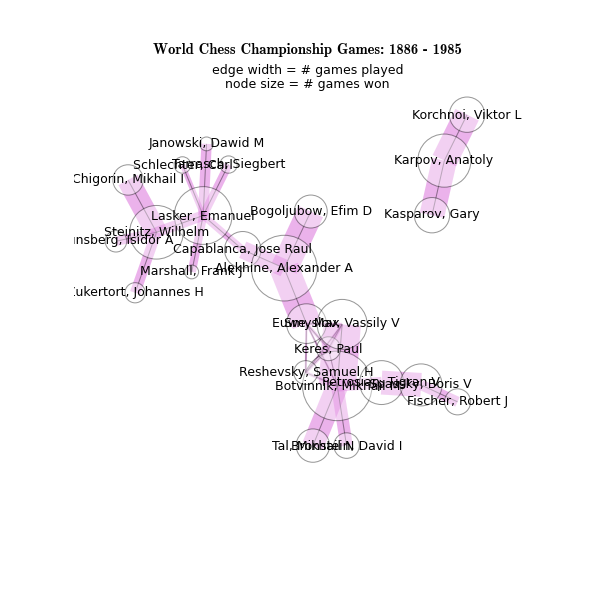Warning

This documents an unmaintained version of NetworkX. Please upgrade to a maintained version and see the current NetworkX documentation.

# Chess Masters¶#!/usr/bin/env python

"""
An example of the MultiDiGraph clas

The function chess_pgn_graph reads a collection of chess
matches stored in the specified PGN file
(PGN ="Portable Game Notation")
Here the (compressed) default file ---
chess_masters_WCC.pgn.bz2 ---
contains all 685 World Chess Championship matches
from 1886 - 1985.

The chess_pgn_graph() function returns a MultiDiGraph
with multiple edges. Each node is
the last name of a chess master. Each edge is directed
from white to black and contains selected game info.

The key statement in chess_pgn_graph below is
where game_info is a dict describing each game.

"""
#    Copyright (C) 2006-2010 by
#    Aric Hagberg <hagberg@lanl.gov>
#    Dan Schult <dschult@colgate.edu>
#    Pieter Swart <swart@lanl.gov>

import networkx as nx

# tag names specifying what game info should be
# stored in the dict on each digraph edge
game_details=["Event",
"Date",
"Result",
"ECO",
"Site"]

def chess_pgn_graph(pgn_file="chess_masters_WCC.pgn.bz2"):
"""Read chess games in pgn format in pgn_file.

Filenames ending in .gz or .bz2 will be uncompressed.

Return the MultiDiGraph of players connected by a chess game.
Edges contain game data in a dict.

"""
import bz2
G=nx.MultiDiGraph()
game={}
datafile = bz2.BZ2File(pgn_file)
lines = (line.decode().rstrip('\r\n') for line in datafile)
for line in lines:
if line.startswith('['):
tag,value=line[1:-1].split(' ',1)
game[str(tag)]=value.strip('"')
else:
# empty line after tag set indicates
# we finished reading game info
if game:
white=game.pop('White')
black=game.pop('Black')
game={}
return G

if __name__ == '__main__':
import networkx as nx

G=chess_pgn_graph()

ngames=G.number_of_edges()
nplayers=G.number_of_nodes()

print("Loaded %d chess games between %d players\n"\
% (ngames,nplayers))

# identify connected components
# of the undirected version
Gcc=list(nx.connected_component_subgraphs(G.to_undirected()))
if len(Gcc)>1:
print("Note the disconnected component consisting of:")
print(Gcc.nodes())

# find all games with B97 opening (as described in ECO)
openings=set([game_info['ECO']
for (white,black,game_info) in G.edges(data=True)])
print("\nFrom a total of %d different openings,"%len(openings))
print('the following games used the Sicilian opening')
print('with the Najdorff 7...Qb6 "Poisoned Pawn" variation.\n')

for (white,black,game_info) in G.edges(data=True):
if game_info['ECO']=='B97':
print(white,"vs",black)
for k,v in game_info.items():
print("   ",k,": ",v)
print("\n")

try:
import matplotlib.pyplot as plt
except ImportError:
import sys
print("Matplotlib needed for drawing. Skipping")
sys.exit(0)

# make new undirected graph H without multi-edges
H=nx.Graph(G)

# edge width is proportional number of games played
edgewidth=[]
for (u,v,d) in H.edges(data=True):
edgewidth.append(len(G.get_edge_data(u,v)))

# node size is proportional to number of games won
wins=dict.fromkeys(G.nodes(),0.0)
for (u,v,d) in G.edges(data=True):
r=d['Result'].split('-')
if r=='1':
wins[u]+=1.0
elif r=='1/2':
wins[u]+=0.5
wins[v]+=0.5
else:
wins[v]+=1.0
try:
pos=nx.graphviz_layout(H)
except:
pos=nx.spring_layout(H,iterations=20)

plt.rcParams['text.usetex'] = False
plt.figure(figsize=(8,8))
nx.draw_networkx_edges(H,pos,alpha=0.3,width=edgewidth, edge_color='m')
nodesize=[wins[v]*50 for v in H]
nx.draw_networkx_nodes(H,pos,node_size=nodesize,node_color='w',alpha=0.4)
nx.draw_networkx_edges(H,pos,alpha=0.4,node_size=0,width=1,edge_color='k')
nx.draw_networkx_labels(H,pos,fontsize=14)
font = {'fontname'   : 'Helvetica',
'color'      : 'k',
'fontweight' : 'bold',
'fontsize'   : 14}
plt.title("World Chess Championship Games: 1886 - 1985", font)

# change font and write text (using data coordinates)
font = {'fontname'   : 'Helvetica',
'color'      : 'r',
'fontweight' : 'bold',
'fontsize'   : 14}

plt.text(0.5, 0.97, "edge width = # games played",
horizontalalignment='center',
transform=plt.gca().transAxes)
plt.text(0.5, 0.94,  "node size = # games won",
horizontalalignment='center',
transform=plt.gca().transAxes)

plt.axis('off')
plt.savefig("chess_masters.png",dpi=75)
print("Wrote chess_masters.png")
plt.show() # display